Refer to our Texas Go Math Grade 6 Answer Key Pdf to score good marks in the exams. Test yourself by practicing the problems from Texas Go Math Grade 6 Module 13 Quiz Answer Key.

13.1 Writing Inequalities

Write an inequality to represent each situation, then graph the solutions.

Question 1.
There are fewer than 8 gallons of gas in the tank. __________________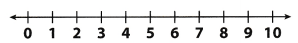Draw a solid circle at 8 to show that 8 is not a solution.
Shade the number line to the left of 8 to show that numbers less than 8 are solutions.
Substitute 7 for g.
7 < 8; 7 is less than 8, so 7 is a solution
Graph the solution on a number line.Question 2.
There are at least 3 pieces of gum left in the pack. __________________Because here we have “at least 3″, the required inequality would be graphed on the following picture.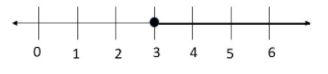The inequality which describes given situation would be x ≥ 3.

Question 3.
The valley was at least 4 feet below sea level. ______________________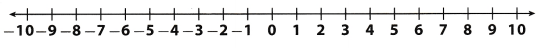Because here we have “at least 4″, the required inequality would be graphed on the following picture.The inequality which describes given situation would be x ≥ 4.

Solve each inequality. Graph the solution.

Question 4.
c – 28 > -32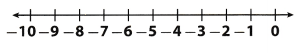We will add 28 to both sides in order to solve this inequality and get:
c > -4
Now, we will graph the solution:Now we will check our solution substituting some value from the set of solutions we got in originaL inequation. We will substitute 0 for c and get:
0 – 28 > -32
-28 > -32
So, the inequality is true.
c <> -4

Question 5.
v + 17 ≤ 20.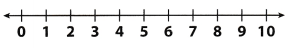In order to solve given inequality, we need to subtract 17 from both sides and get:
v ≤ 3
Now, we will graph the solution:Question 6.
Today’s high temperature of 80°F is at least 16° warmer than yesterday’s high temperature. What was yesterday’s high temperature?
Let x represent yesterday’s high temperature. According to all informations, we get the following inequality:
80 – x ≥ 16
We need to subtract 80 from both sides and get:
-x ≥ -64
Next step is to multiply both sides by -1. -1 is negative number, so, we need to reverse the inequality symbol for the statement to still be true.
(-x) (-1) ≤ (-64) (-1)
x ≤ 64
So, yesterday’s high temperature was at most 64°F.

13.3, 13.4 Multiplication and Division inequalities

Solve each inequality. Graph the solution.

Question 7.
7f ≤ 35First step is to divide both sides by 7.
$$\frac{7f}{7}$$ ≤ $$\frac{35}{7}$$
f ≤ 5
Now, we will graph the solution: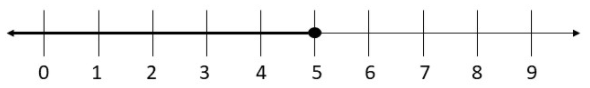Now we will check the solution by substituting a soLution from the shaded part of the graph into the original inequality.
We will substitute 2 for f and get:
7 ∙ 2 ≤ 35
14 ≤ 35
So, the inequality is true.
f ≤ 5

Question 8.
$$\frac{a}{2}$$ < 4First step is to multiply by 2.
2($$\frac{a}{2}$$) < 4(2)
a < 8
Now, we will graph the solution:Now, we will check the solution by substituting a solution from the shaded part of the graph into the original inequality. We will substitute 4 for a and get:
$$\frac{4}{2}$$ < 4
2 < 4
So, the inequality is true.
a < 8

Question 9.
-25g ≥ 150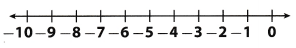First step is to divide both sides by -25. -25 is negative number, so we need to reverse the inequality symbol for the statement to still be true.
$$\frac{-25g}{-25}$$ ≤ $$\frac{150}{-25}$$
g ≤ -6
Now, we will graph the solution: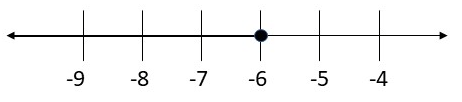Now we will check the solution by substituting a solution from the shaded part of the graph into the original inequality.
We will substitute -8 for g and get:
-25(-8) ≥ 150
200 ≥ 150
So, the inequality is true.
g ≤ -6

Question 10.
$$\frac{k}{-3}$$ < 3First step is to multiply by -3. -3 is negative number, so we need to reverse the inequality symbol for the statement to still be true.
$$\frac{k}{-3}$$(-3) > 3(-3)
k > -9
Now, we will graph the solution:Now we will check the solution by substituting a solution from the shaded part of the graph into the original inequality.
So, we will substitute 0 for k and get:
$$\frac{0}{-3}$$ < 3
0 < 3 So, the inequality is true. k > -9

Texas Go Math Grade 6 Module 13 Mixed Review Texas Test Prep Answer Key

Selected Response

Question 1.
Em saves at least 20% of what she earns each week. If she earns $140 each week for 4 weeks, which inequality describes the total amount she saves? (A) t > 112 (B) t ≥ 112 (C) t < 28 (D) t ≤ 28 Answer: (D) t ≤ 28 Explanation: First we will calculate how much is 20% of 140. So we will multiply 140 by $$\frac{20}{100}$$ = $$\frac{1}{5}$$. 140 x $$\frac{1}{5}$$ = $$\frac{140 \times 1}{1 \times 5}$$ = 28 So, if t represent Em’s savings, the inequality which describes given situation is: t ≤ 28 Question 2. Which number line represents the inequality r > 6?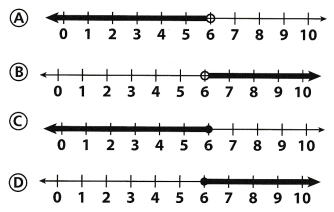Answer: (B) Explanation: This inequality represents numbers on the right side of 6 and because there is symbol >, the circle at 6 is not full. So, conclusion is that number line which represents the inequality r > 6 is B. Question 3. For which inequality below is z = 3 a solution? (A) z + 5 ≥ 9 (B) z + 5 > 9 (C) z + 5 ≤ 8 (D) z + 5 < 8 Answer: (C) z + 5 ≤ 8 Explanation: Solution of inequality at C is the following: z ≤ 3 This solution includes 3, so, for this inequality, z = 3 is a solution. Question 4. What is the solution to the inequality -6x < -18? (A) x > 3 (B) x < 3 (C) x ≥ 3 (D) x ≤ 3 Answer: (A) x > 3 Explanation: In order to solve this inequality, we need to divide both sides by -6. -6 is negative number, so, we need to reverse the inequality symbol for the statement to still be true. $$\frac{-6x}{-6}$$ > $$\frac{-18}{-6}$$ x > 3 Question 5. The number line below represents the solution to which inequality?(A) $$\frac{m}{4}$$ > 2.2 (B) 2m < 17.6 (C) $$\frac{m}{3}$$ > 2.5 (D) 5m > 40 Answer: (A) $$\frac{m}{4}$$ > 2.2 Explanation: On given number line there are numbers right from 8.8 and 9 is not included. That is actually m > 8.8. We can notice that this is soLution of inequality at A. 4($$\frac{m}{4}$$) > (2.2) (4) m > 8.8 Question 6. Which number line shows the solution to w – 2 ≤ 8?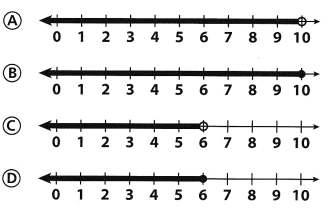Answer: (B) Explanation: In order to solve given inequality, we need to add 2 to both sides: w ≤ 10 Solution we got appropriate to B. Gridded Response Question 7. Hank needs to save at least$150 to ride the bus to his grandparent’s home, If he saves \$12 a week, what is the least number of weeks he needs to save?$$\frac{12x}{12}$$ ≥ $$\frac{150}{12}$$